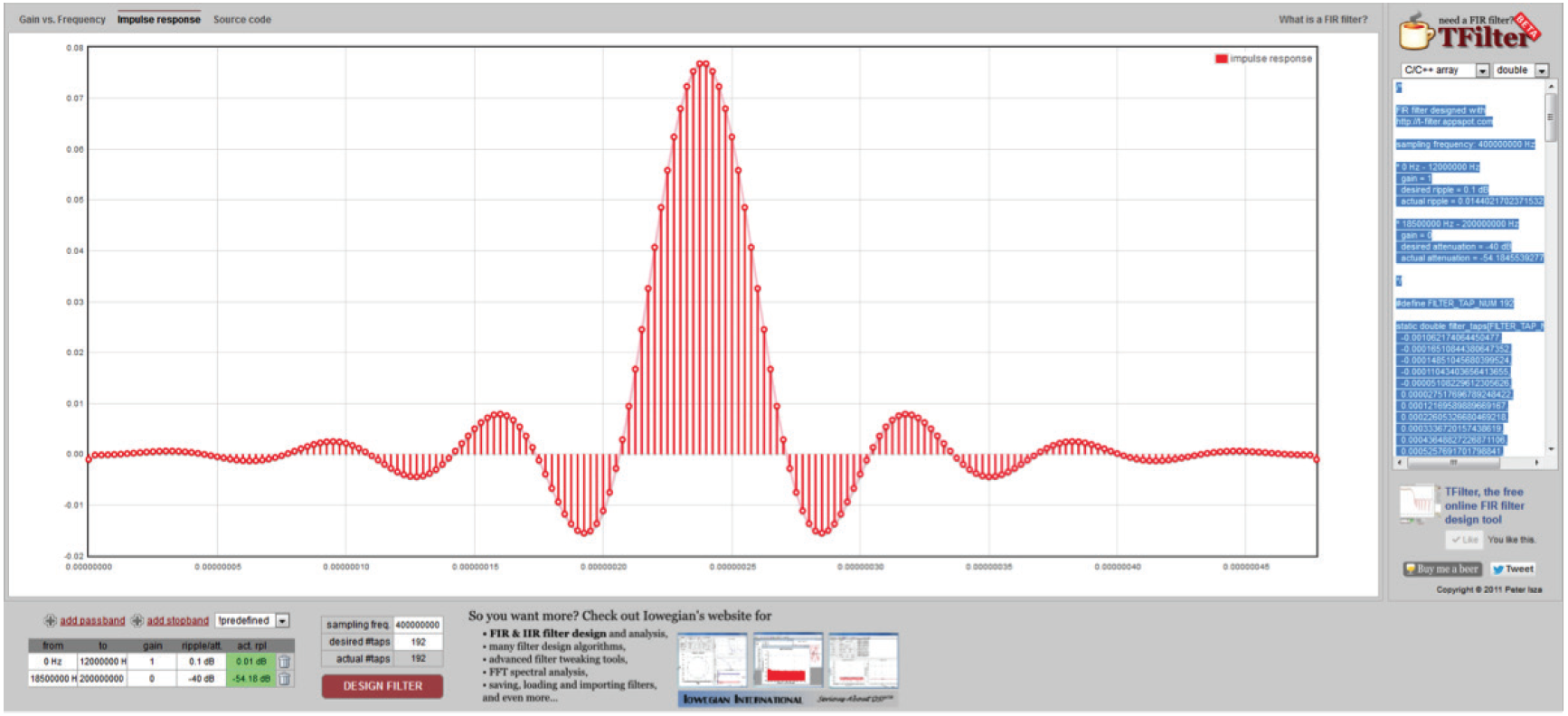# Modeling and Synthesizing Large Ratio Rate AdaptersSometimes engineers are called upon to design sample rate adapters with ratios that do not fit convenient integer rates of decimation or interpolation.

Decimating or interpolating by ratios of less than two can lead to large ratios of required interpolation and decimation that would be inefficient if implemented as cascaded filters.This document examines an algorithmic solution for minimal hardware and efficient implementation of such large ratio rate adapters.

### What you get

The zipped folder contains a design source code example with a simple testbench and an Application Note detailing the design of a 37/50 rate adaptor that can be applied to any large ratio rate conversion.

• downconverter.cpp - The core implementation in C++
• downconverter.h - A header file for the implementation
• linear.cpp - A C++ implementation of linear interpolation with no filtering
• script.tcl - A script for Catapult®HLS
• tb_downconverter.cpp - A testbench for the implementation (for C++ testing and reuse with the RTL)
• Application Note - Modeling and Synthesizing Large Ratio Rate Adapters